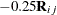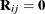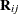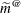### Example 41.8 Adjusted Covariance Matrices of Fixed Effects

The following data are from Pothoff and Roy (1964) and consist of growth measurements for 11 girls and 16 boys at ages 8, 10, 12, and 14. Some of the observations are suspect (for example, the third observation for person 20); however, all of the data are used here for comparison purposes.

```data pr;
input child gender\$ y1 y2 y3 y4;
array yy y1-y4;
do time=1 to 4;
age = time*2 + 6;
y   = yy{time};
output;
end;
drop y1-y4;
datalines;
1   F   21.0    20.0    21.5    23.0
2   F   21.0    21.5    24.0    25.5
3   F   20.5    24.0    24.5    26.0
4   F   23.5    24.5    25.0    26.5
5   F   21.5    23.0    22.5    23.5
6   F   20.0    21.0    21.0    22.5
7   F   21.5    22.5    23.0    25.0
8   F   23.0    23.0    23.5    24.0
9   F   20.0    21.0    22.0    21.5
10   F   16.5    19.0    19.0    19.5
11   F   24.5    25.0    28.0    28.0
12   M   26.0    25.0    29.0    31.0
13   M   21.5    22.5    23.0    26.5
14   M   23.0    22.5    24.0    27.5
15   M   25.5    27.5    26.5    27.0
16   M   20.0    23.5    22.5    26.0
17   M   24.5    25.5    27.0    28.5
18   M   22.0    22.0    24.5    26.5
19   M   24.0    21.5    24.5    25.5
20   M   23.0    20.5    31.0    26.0
21   M   27.5    28.0    31.0    31.5
22   M   23.0    23.0    23.5    25.0
23   M   21.5    23.5    24.0    28.0
24   M   17.0    24.5    26.0    29.5
25   M   22.5    25.5    25.5    26.0
26   M   23.0    24.5    26.0    30.0
27   M   22.0    21.5    23.5    25.0
;
```

Jennrich and Schluchter (1986) analyze these data with various models for the fixed effects and the covariance structure. The strategy here is to fit a growth curve model for the boys and girls and to account for subject-to-subject variation through G-side random effects. In addition, serial correlation among the observations within each child is accounted for by a time series process. The data are assumed to be Gaussian, and their –2 restricted log likelihood is minimized to estimate the model parameters.

The following statements fit a mixed model in which a separate growth curve is assumed for each gender:

```proc glimmix data=pr;
class child gender time;
model y = gender age gender*age / covb(details) ddfm=kr;
random intercept age / type=chol sub=child;
random time / subject=child type=ar(1) residual;
ods select ModelInfo CovB CovBModelBased CovBDetails;
run;
```

The growth curve for an individual child differs from the gender-specific trend because of a random intercept and a random slope. The two G-side random effects are assumed to be correlated. Their unstructured covariance matrix is parameterized in terms of the Cholesky root to guarantee a positive (semi-)definite estimate. An AR(1) covariance structure is modeled for the observations over time for each child. Notice the RESIDUAL option in the second RANDOM statement. It identifies this as an R-side random effect.

The DDFM=KR option requests that the covariance matrix of the fixed-effect parameter estimates and denominator degrees of freedom for t and F tests are determined according to Kenward and Roger (1997). This is reflected in the Model Information table (Output 41.8.1).

Output 41.8.1: Model Information with DDFM=KR

The GLIMMIX Procedure

Model Information
Data Set WORK.PR
Response Variable y
Response Distribution Gaussian
Variance Function Default
Variance Matrix Blocked By child
Estimation Technique Restricted Maximum Likelihood
Degrees of Freedom Method Kenward-Roger

The COVB option in the MODEL statement requests that the covariance matrix used for inference about fixed effects in this model is displayed; this is the Kenward-Roger-adjusted covariance matrix. The DETAILS suboption requests that the unadjusted covariance matrix is also displayed (Output 41.8.2). In addition, a table of diagnostic measures for the covariance matrices is produced.

Output 41.8.2: Model-Based and Adjusted Covariance Matrix

Model Based Covariance Matrix for Fixed Effects (Unadjusted)
Effect gender Row Col1 Col2 Col3 Col4 Col5 Col6
Intercept   1 0.9969 -0.9969   -0.07620 0.07620
gender F 2 -0.9969 2.4470   0.07620 -0.1870
gender M 3
age   4 -0.07620 0.07620   0.007581 -0.00758
age*gender F 5 0.07620 -0.1870   -0.00758 0.01861
age*gender M 6

Covariance Matrix for Fixed Effects
Effect gender Row Col1 Col2 Col3 Col4 Col5 Col6
Intercept   1 0.9724 -0.9724   -0.07412 0.07412
gender F 2 -0.9724 2.3868   0.07412 -0.1819
gender M 3
age   4 -0.07412 0.07412   0.007256 -0.00726
age*gender F 5 0.07412 -0.1819   -0.00726 0.01781
age*gender M 6

Diagnostics for Covariance Matrices of Fixed Effects
Dimensions Rows 6 6
Non-zero entries 16 16

Summaries Trace 3.4701 3.3843
Log determinant -11.95 -12.17

Eigenvalues > 0 4 4
= 0 2 2
max abs 2.972 2.8988
min abs non-zero 0.0009 0.0008
Condition number 3467.8 3698.2

Norms Frobenius 3.0124 2.9382
Infinity 3.7072 3.6153

Comparisons Concordance correlation   0.9979
Discrepancy function   0.0084
Frobenius norm of difference   0.0742

The Diagnostics for Covariance Matrices table in Output 41.8.2 consists of several sections. The trace and log determinant of covariance matrices are general scalar summaries that are sometimes used in direct comparisons, or in formulating further statistics, such as the difference of log determinants. The trace simply represents the sum of the variances of all fixed-effects parameters.

The two matrices have the same number of positive and zero eigenvalues; hence they are of the same rank. There are no negative eigenvalues; hence the matrices are positive semi-definite.

The Comparisons section of the table provides several statistics that set the matrices in relationship. The statistics enable you to assess the extent to which the adjustment affected the model-based matrix. If the two matrices are identical, the concordance correlation equals 1, the discrepancy function and the Frobenius norm of the differences equal 0, and the trace of the adjusted and the (generalized) inverse of the model-based matrix equals the rank. See the section Exploring and Comparing Covariance Matrices for computational details regarding these statistics. With increasing discrepancy between the matrices, the difference norm and discrepancy function increase, the concordance correlation falls below 1, and the trace deviates from the rank. In this particular example, there is strong agreement between the two matrices; the adjustment to the covariance matrix associated with DDFM=KR is only slight. It is noteworthy, however, that the trace of the adjusted covariance matrix falls short of the trace of the unadjusted one. Indeed, from Output 41.8.2 you can see that the diagonal elements of the adjusted covariance matrices are uniformly smaller than those of the model-based covariance matrix.

Standard error shrinkage for the Kenward-Roger covariance adjustment is due to the termin equation (3) of Kenward and Roger (1997), which is nonzero for covariance structures with second derivatives, such as the TYPE=ANTE(1), TYPE=AR(1), TYPE=ARH(1), TYPE=ARMA(1,1), TYPE=CHOL, TYPE=CSH, TYPE=FA0(q), TYPE=TOEPH, and TYPE=UNR structures and all TYPE=SP() structures.

For covariance structures that are linear in the parameters,. You can add the FIRSTORDER suboption to the DDFM=KR option to request that second derivative matricesare excluded from computing the covariance matrix adjustment. The resulting covariance adjustment is that of Kackar and Harville (1984) and Harville and Jeske (1992). This estimator is denoted asin Harville and Jeske (1992) and is referred to there as the Prasad-Rao estimator after related work by Prasad and Rao (1990). This standard error adjustment is guaranteed to be positive (semi-)definite. The following statements fit the model with the Kackar-Harville-Jeske estimator and compare model-based and adjusted covariance matrices:

```proc glimmix data=pr;
class child gender time;
model y = gender age gender*age / covb(details)
ddfm=kr(firstorder);
random intercept age / type=chol sub=child;
random time / subject=child type=ar(1) residual;
ods select ModelInfo CovB CovBDetails;
run;
```

The standard error adjustment is reflected in the Model Information table (Output 41.8.3).

Output 41.8.3: Model Information with DDFM=KR(FIRSTORDER)

The GLIMMIX Procedure

Model Information
Data Set WORK.PR
Response Variable y
Response Distribution Gaussian
Variance Function Default
Variance Matrix Blocked By child
Estimation Technique Restricted Maximum Likelihood
Degrees of Freedom Method Kenward-Roger

Output 41.8.4 displays the adjusted covariance matrix. Notice that the elements of this matrix, in particular the diagonal elements, are larger in absolute value than those of the model-based estimator (Output 41.8.2).

Output 41.8.4: Adjusted Covariance Matrix and Comparison to Model-Based Estimator

Covariance Matrix for Fixed Effects
Effect gender Row Col1 Col2 Col3 Col4 Col5 Col6
Intercept   1 1.0122 -1.0122   -0.07758 0.07758
gender F 2 -1.0122 2.4845   0.07758 -0.1904
gender M 3
age   4 -0.07758 0.07758   0.007706 -0.00771
age*gender F 5 0.07758 -0.1904   -0.00771 0.01891
age*gender M 6

Diagnostics for Covariance Matrices of Fixed Effects
Dimensions Rows 6 6
Non-zero entries 16 16

Summaries Trace 3.4701 3.5234
Log determinant -11.95 -11.91

Eigenvalues > 0 4 4
= 0 2 2
max abs 2.972 3.0176
min abs non-zero 0.0009 0.0009
Condition number 3467.8 3513.4

Norms Frobenius 3.0124 3.0587
Infinity 3.7072 3.7647

Comparisons Concordance correlation   0.9999
Discrepancy function   0.0003
Frobenius norm of difference   0.0463